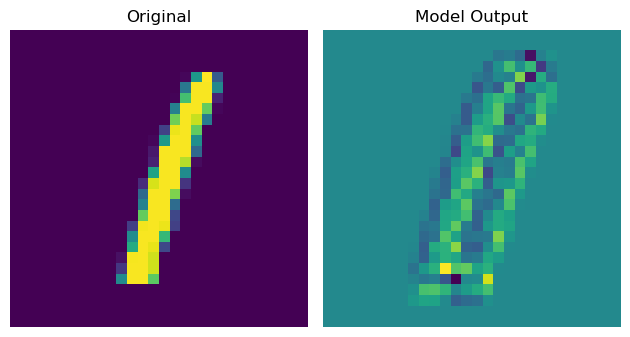# Custom Conv2D implementation from scratch not learning correctly

I have been attempting to implement my own Conv2D layer to better understand how it operates but I’m having some issues. I am using a simple CNN based auto encoder as my baseline training on the MNIST dataset. I am not using a complicated encoder/decoder, just a single conv2d layer. Here is my model:

``````class AutoEncoder(torch.nn.Module):
def __init__(self):
super().__init__()
self.enc = torch.nn.Conv2d(1, 10, 3, stride=1)
self.dec = torch.nn.ConvTranspose2d(10, 1, 3, stride=1)

def forward(self, x):
return self.dec(self.enc(x))
``````

As you can see, I am using 10 filters, a window size of 3, and a stride of 1. The results I am getting from this are really good! After two epochs of training on the MNIST, I get: `test_loss 0.0009646228281781077` which is not unexpected. I wanted to post a photo of the expected next to the model output, but due to the forum limitations (since I am new) I can only post one photo. End of the day, the original image and the reconstruction after going through the model is nearly identical.

Moving on to my custom implementation. I am using fold and unfold to slice the image and reconstruct it. Below is the bulk of my conv code. I am using hardcoded image slices for MNIST and reshaping the fold/unfold inputs/outputs for this to make it simpler to understand quickly.

``````im = next(iter(trainloader)) #just get a single batch of MNIST train set in format b c h w
encoder_weights = torch.randn(10, 1, 3, 3, requires_grad=True) #filter, channel, height, width
decoder_weights = torch.randn(1, 10, 3, 3, requires_grad=True) #filter, channel, height, width
unfolded = F.unfold(im, 3, 1).view(-1, 1, 3, 3, 26, 26) #batch, channel*prod(kernel*[2 dims]), height*width (out) -> batch, channel, kernel, kernel, height, width
enc = torch.einsum('bcdehw, fcde -> bfhw', unfolded, encoder_weights).unsqueeze(2).unsqueeze(2) #batch, channel (filter out), 1, 1 height, width
dec = torch.einsum('bcdehw, fcde -> bfdehw', enc, decoder_weights).view(-1, 9, 676) #batch, channel (filter out), kernel, kernel, height, width -> batch, channel*prod(kernel*[2 dims]), height*width (out)
folded = F.fold(dec, im.shape[-2:], 3, 1)
``````

My output after training looks like the following:Clearly this isn’t the intended output and only gets worst as training goes on. Does anyone have any direction I could look to solve this issue? Just for completeness, I am using PyTorch Lightning and my custom implementation is listed below.

``````class AutoEncoder(pl.LightningModule):
def __init__(self):
super().__init__()

self.encoder_weights = t.nn.Parameter(t.randn(10, 1, 3, 3, requires_grad=True)) #filter, channel, height, width
self.decoder_weights = t.nn.Parameter(t.randn(1, 10, 3, 3, requires_grad=True)) #filter, channel, height, width

def forward(self, x):
unfolded = F.unfold(x, 3, 1).view(-1, 1, 3, 3, 26, 26) #batch, channel*prod(kernel*[2 dims]), height*width (out) -> batch, channel, kernel, kernel, height, width
enc = t.einsum('bcdehw, fcde -> bfhw', unfolded, self.encoder_weights).unsqueeze(2).unsqueeze(2) #batch, channel (filter out), 1, 1 height, width
dec = t.einsum('bcdehw, fcde -> bfdehw', enc, self.decoder_weights).view(-1, 9, 676) #batch, channel (filter out), kernel, kernel, height, width -> batch, channel*prod(kernel*[2 dims]), height*width (out)
folded = F.fold(dec, x.shape[-2:], 3, 1)
return folded

def training_step(self, batch, batch_idx):
x, y = batch
x_hat = self.forward(x)
loss = F.mse_loss(x_hat, x)
self.log("train_loss", loss)
return loss

def configure_optimizers(self):
If anyone else runs into this issue, I ended up using this immediately after declaring my `encoder_weights` and `decoder_weights`:
``````torch.nn.init.kaiming_uniform_(self.encoder_weights, a=np.sqrt(9))